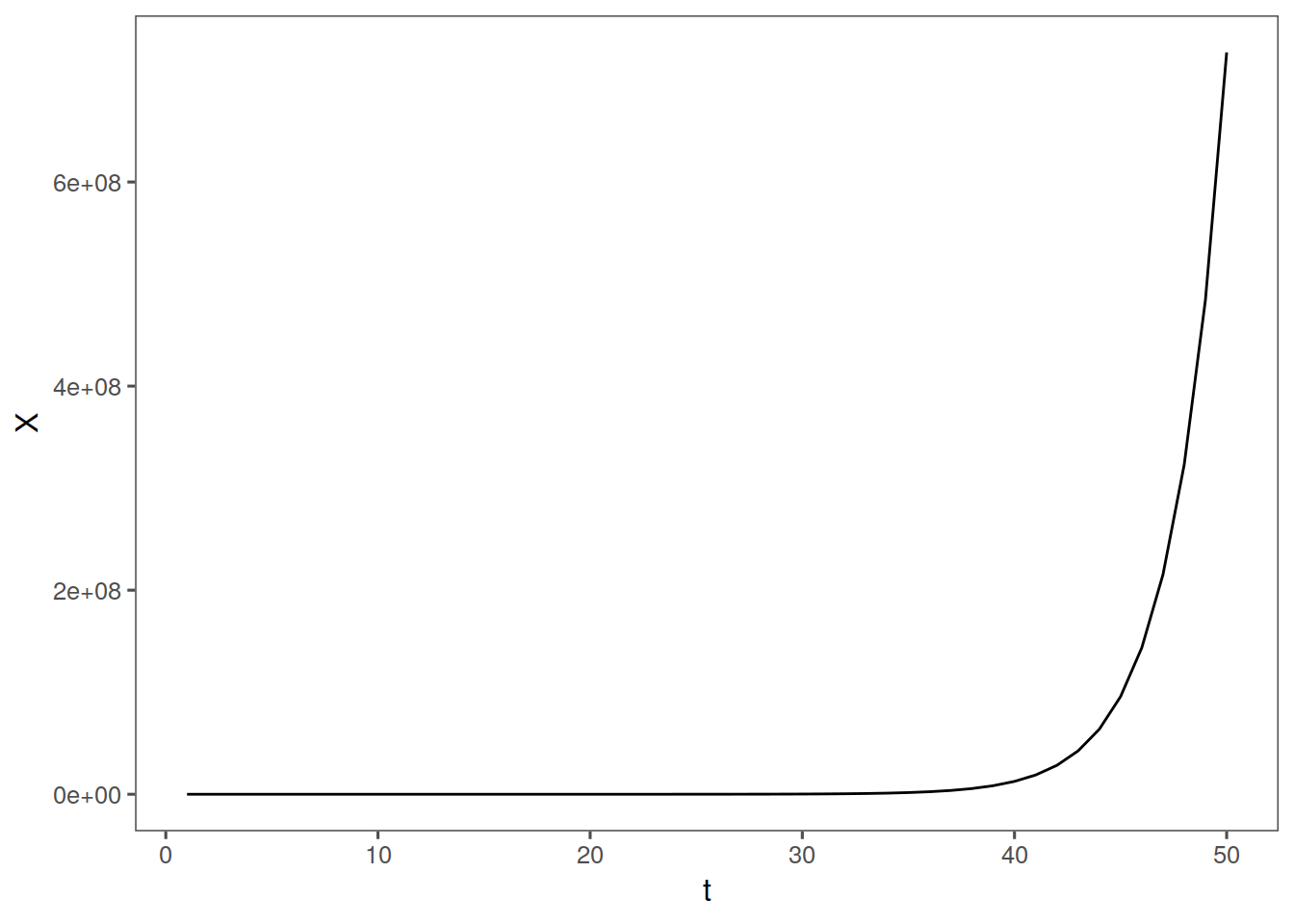# Unit 10 GLPs

a GLP is a linear filter with a white noise input

We take white noise input and put it through a filter and get something that isnt white noise.

$\sum_{j=0}^\infty \psi_j a_{t-j} = X_t - \mu$

GLP’s are an infinite sum of white noise terms. This might be weird now but later on this concept will return.

The $$\psi_j$$’s are called psi weights, these will be useful again later. AR, MA, and ARMA are all special cases of GLP’s, and will be useful when we study confidence intervals.

## 10.1 AR(1) Intro

AR(p) in which p = 1

We will go through well known forms of the AR(1) model.

$X_t = \beta + \phi_1 X_{t-1} + a_t$

$\beta = (1-\phi_1)\mu$

Beta is moving average constant

We are saying that the vluse of x depends on some constant, the previous value of x, and some random white noise. Looks a lot like regression except there is a wierd variable

we can rewirte tis as

$X_t = (1-\phi_1)\mu + \phi_1 X_{t-1} + a_t$

An are 1 process is stationary iff the magnitude of $$\phi_1$$ is less than 1

We will deal with this a lot in the near future

### 10.1.1 AR(1) math zone

$E\left[ X_t \right] = \mu ?$ $E \left[ X_t \right] =E \left[ 1-\phi_1 \mu \right] + E \left[ \phi_1 X_{t-1} \right] + E[a_t]$

We can rewrite this as $E \left[ X_t \right] = 1-\phi_1 \mu + \phi_1E \left[ X_{t} \right] + 0$

$E \left[ X_t \right] ( 1-\phi_1) = 1-\phi_1 \mu$ $E\left[ X_t \right] = \mu$

Mean does not depend on T

The variance also does not, and if phi1 is less than one variance is finite

$\sigma_X^2 = \frac{\sigma_a^2}{1-\phi_1^2}$

and rhok is phi1 to the k

$\rho_k = \phi_1^k, k \geq 0$

Spectral Density of AR(1) also does not depend on time, it just monotonically increases or decreases depending on phi1:

$S_X(f) = \frac{\sigma_a^2}{\sigma_X^2} \left( \frac{1}{\mid 1 - \phi_1 e^{-2\pi i f}\mid^2} \right)$

### 10.1.2 the zero mean form of AR1

with zero mean, $X_t = \phi_1 X_{t-1} + a_t$ OR $X_t- \phi_1 X_{t-1}$A

### 10.1.3 AR1 with positive phi

remember we have $\rho_k = \phi_1^k$

With positive $$\phi_1$$, we have:

realizations are wandering and aperiodic

Autocorrelations are damped exponentials

Spectral density Peaks at zero.

Higher values = stronger versions of these characterstics

### 10.1.4 AR1 with negative phi

We have:

realization are oscillating

Autocorrelations are damped and alternating(negative to a power you fool)

Spectral density* has a peak at f = 0.5 (cycle length of 2)

Higher magnitude = stronger characteristics

### 10.1.5 Nonstationary

if phi = 1 or phi> 1, we have that realizations are from a nonstationary process. with it equal to one, it looks ok, and is actualy a special ARIMA model. WIth it > 1, we have crazy explosive realizations. Check this out:

nonstarma <- function(n, phi) {
x <- rep(0, n)
a <- rnorm(n)
x[1:n] <- 0
for (k in 2:n) {
x[k] = phi * x[k - 1] + a[k]
}
tplot(x)
}

nonstarma(n = 50, phi = 1.5) + th